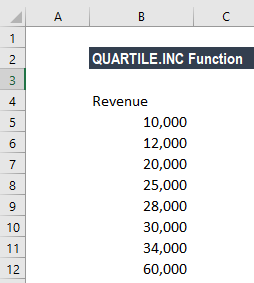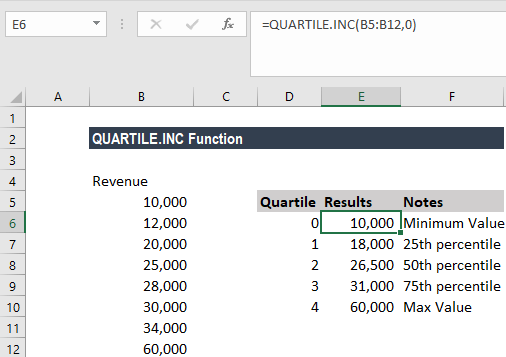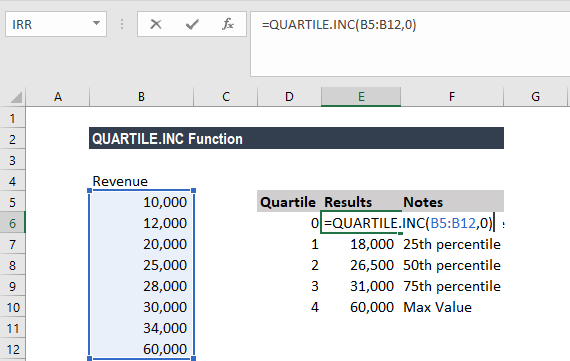# QUARTILE.INC Function

Returns the quartile of a given data set based on percentile values from 0.1 inclusive

## What is the QUARTILE.INC Function?

The QUARTILE.INC Function is categorized under Excel  Statistical functions. It will return the quartile of a given data set based on percentile values from 0.1, inclusive. The function bases its calculation on a percentile range of 0 to 1, inclusive.

As a financial analyst, QUARTILE.INC can be used to find out the minimum and maximum revenue for a quarter for a given population. The function can also be used in revenue analysis.

### Formula

=QUARTILE.INC(array,quart)

The QUARTILE.INC function uses the following arguments:

1. Array (required argument) – This is the array or cell range of data values for which we want the quartile value.
2. Quart (required argument) – It indicates which value shall be returned. It is an integer between 0 and 4, representing the required quartile.

QUARTILE.INC is closely related to the PERCENTILE.INC function. It accepts five values for the quart argument, as shown in the table below:

QuartReturn value
0Min value
1First quartile – 25th percentile
2Median value – 50th percentile
3Third quartile – 75th percentile
4Max value

### How to use the QUARTILE.INC Function in Excel?

To understand the uses of the QUARTILE.INC function, let’s consider an example:

#### Example 1

Suppose we are given the following revenue figures:QUARTILE.INC can return the minimum value, first quartile, second quartile, third quartile, and max value. The function accepts five values for the quart argument, as shown below:The formula used was:Similarly, we used 1 for the 25th percentile, 2 for the 50th percentile, 3 for the 75th percentile and 4 for the Max value.

### Few notes about the QUARTILE.INC Function

1. #NUM! error – Occurs if either:
• The given value of quart is < 0 or > 4; or
• The given array is empty.
2. #VALUE! error – Occurs if the given value of quart is non-numeric.
3. From Excel 2010, the QUARTILE.EXC and QUARTILE.INC functions would replace the QUARTILE function, which is now classified as a compatibility function.
4. The QUARTILE.INC and QUARTILE.EXC functions both find a requested quartile of a supplied data set. The difference between these two functions is that QUARTILE.INC bases its calculation on a percentile range of 0 to 1 inclusive, whereas QUARTILE.EXC bases its calculation on a percentile range of 0 to 1 exclusive.
5. If the requested quartile falls between two of the values in the supplied array, MS Excel interpolates between these values to calculate the quartile value.

Thanks for reading CFI’s guide to the Excel QUARTILE.INC function. By taking the time to learn and master these Excel functions, you’ll significantly speed up your financial analysis. To learn more, check out these additional CFI resources:

• Excel Functions for Finance